HOMEWORK SOLUTION

HW #4

GEOTECHNICAL ENGINEERING I

(Question no. 4.3-, 4.6, 4.11……Al-Khafaji textbook)4.3       a. Estimate optimum gd corresponding to optimum w (water content)

b. What is the range of w if % compaction = 95%

Solution:

See graph 1. :

a. Optimum gd = 125 pcf

Optimum w = 16%...ans

b. 95% =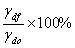gdf = 95% x 125 pcf = 118.75 pcf

plot the graph we get the range of w = 9.5% - 21.5% ……….ans.

4.4      Vs = 2,000,000 yards3 = 54 x 106 ft3    (for embankment)   note: 1 yd3=27ft3

gm for embankment  = 125 pcf

w for embankment  = 15.8 %

Select one of the pit (borrowing soil) which is the minimum cost.

 pit gm w cost/yd3 A 110.5 12.2 6.2 B 107.4 15.0 5.0 C 90 27.4 3.5

Solution:

Soil mass need for embankment   Ws = gd x Vs =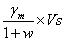Ws =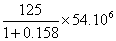= 5.83 x 106 lb

Pit A.

VsA ==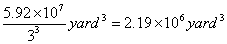costA = VsA x \$6.2 = \$ 13.578 million.

Pit B.

VsA ==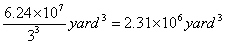costB = VsB x \$5.0 = \$ 11.55 million.

Pit C.

VsA ==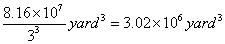costC = VsC x \$3.5 = \$ 10.57 million.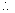The minimum cost is Pit C…………………..ans.

4.11     Volume soil field           = 1162 cm3

Weight of soil in the field (wet)  = 2253 gr

Weight of soil in the field (dry)   = 1910 gr

See graph 2:

Question:

(a)    gdf ?

(b)   wf ?

(c)    % compaction ?

Solution:

(a)    gdf =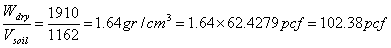ans

(b)   wf =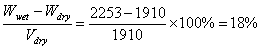……………….ans

(c)    % compaction =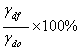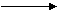gdo                          from graph 2. we obtain

gdo = 119.5 pcf

gdf = 102.38 pcf

% compaction =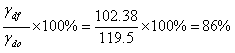…..ans